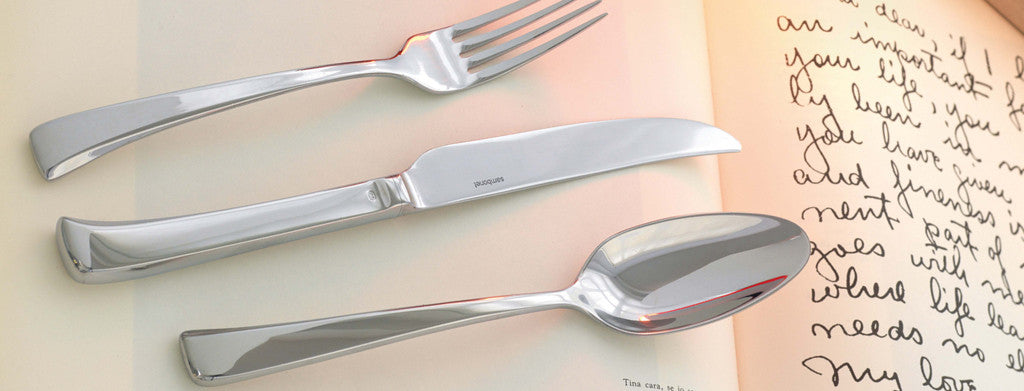Shopping Cart

### Refine

View all

#### Price# Cutlery£5.48 each (ex VAT)£5.59 each (ex VAT)£5.59 each (ex VAT)£6.15 each (ex VAT)£5.48 each (ex VAT)£7.57 each (ex VAT)£5.48 each (ex VAT)£8.70 each (ex VAT)£8.87 each (ex VAT)£6.15 each (ex VAT)£6.26 each (ex VAT)£7.57 each (ex VAT)£9.17£6.15 each (ex VAT)£6.76 each (ex VAT)£5.48 each (ex VAT)£8.70 each (ex VAT)£5.07 each (ex VAT)£6.61 each (ex VAT)£5.80 each (ex VAT)£8.70 each (ex VAT)£7.74 each (ex VAT)£7.74 each (ex VAT)£7.74 each (ex VAT)£6.44 each (ex VAT)£5.68 each (ex VAT)£9.51 each (ex VAT)£7.39 each (ex VAT)£8.69 each (ex VAT)£8.69 each (ex VAT)£0.31 each (ex VAT)£0.55 each (ex VAT)£5.03 each (ex VAT)£9.48 each (ex VAT)£9.48 each (ex VAT)£7.74 each (ex VAT)£7.74 each (ex VAT)£6.15 each (ex VAT)£8.70 each (ex VAT)£8.70 each (ex VAT)£5.80 each (ex VAT)£8.70 each (ex VAT)£8.70 each (ex VAT)£5.52 each (ex VAT)£8.38 each (ex VAT)£8.38 each (ex VAT)£9.17£5.80 each (ex VAT)